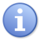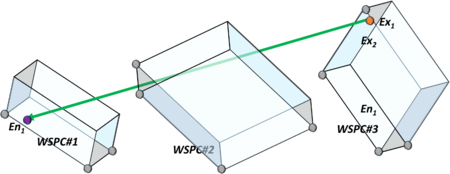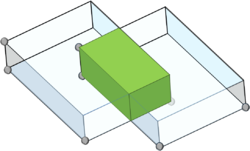# Introduction

This feature limits robot movements inside defined WorkSpaces areas and at specific paths between them. Each WorkSpaces is modeled by users as a rectangular prism in 3D space with entry and exit points, which defined in *.XML file.
Robot is considered to be inside WorkSpaces if its TCP (Tool Center Point) is within the prism and outside WorkSpaces if its TCP is outside the prism.
In case user tries to move the robot outside WorkSpaces using unauthorized path an error will raise and motion won't be started.NOTE Robot segments are not considered

# WorkSpace Properties

WorkSpaces are cuboids defined by its four vertex points:

• Origin (O)
• Width (W)
• Length (L)
• Height (H)

The cuboid can have any orientation in space. The coordinates of the cuboid are given in WorkPiece coordinates of the robot TCP (including tool).
Cuboid parameter defines relative to WorkSpace origin:

• O = #{X,Y,Z,Yaw,Pitch,Roll} - location
• L = O:#{0,WSCPL,0,0,0,0}
• W = O:#{WSCPW,0,0,0,0,0}
• H = O:#{0,0,WSCPH,0,0,0}

When WSPCL = WorkSpace length, WSPCW = WorkSpace width, WSPCH = WorkSpace height. To sets a proper WorkSpace one need to follow these rules:

1. WorkSpaces are grouped in WorkSpace-sets. There will be maximum 16 WorkSpace defined in one WorkSpace-set when each WorkSpace-set belongs to only one robot.
2. WorkSpace will be indexed by unique numbers 1, 2, 3, ...
3. Only active WorkSpace is considered.
4. WorkSpace areas won't be able to edit (change) while robot is enabled (powered-on).
5. WorkSpace can be activated only when the robot is disabled (powered-off).
6. WorkSpace areas won't be active during conveyer tracking.
7. In the first phase, if there are active WorkSpace lined to a robot joint-interpolated motions (MOVE) will be not allowed!
8. Each WorkSpace area will have a set of entry & exit points: (En1,En2, …) and (Ex1,Ex2, …) defining exit and entry paths from one workspace to another.
9. Entry and exit points will be defined only by #{X,Y,Z} relative to the origin (O) of the related working space.
10. Entry and exit points must lie on the surface of the WorkSpace (not inside the WorkSpace).
11. Entry and exit points will be defined with a tolerance radius R meaning that if the robot TCP is at distance less then R from the given point it will be considered at this point. The tolerance will be described by half-sphere of radius R – meaning only the half that is inside given WorkSpace is valid.
12. There will be only one exit and one entry path from one given pair of WorkSpaces
13. User can move the robot from one WorkSpace area to another only if:
1. Movement is straight line movement (MOVES, MOVESKD), other interpolation types like: CIRCLE, MOVE, JOG are not allowed.
2. Movement starts from one of the exit points of the WorkSpace area (Exi) to the entry-point (Enj) of another WorkSpace area.
3. No other movement outside WorkSpace area are allowed.
14. To move a robot to a WorkSpace area From random location without using WorkSpace paths, one need to issuing separate axis movements:
Move A1 10
Move A2 -40 ....
15. Different robots can have different WorkSpace sets.
16. It will be possible to know for each robot in which WorkSpace-index the robot is currently placed.

# Graphic Movement Examples

To understand the movement between WorkSpaces follow the next scenario:
• There are three workspace objects:
• wspc#1
• wspc#2
• wspc#3
• Only the following exit/entry paths between workspaces are allowed:
• wspc#1.Ex1→ wspc#2.En2
• wspc#2.Ex1→ wspc#3.En1
• wspc#3.Ex1→ wspc#1.En1
• wspc#3.Ex2→ wspc#2.En1

It's allowed for entry and exit paths to cross WorkSpaces and other WorkSpaces areas unlimited number of times:In case two WorkSpaces areas have common intersection, robot will be able to move from one to another without limitations:# Initialization

Initialization of one WorkSpace set will be done by reading an XML file with coordinates and entry/exit points linked to a specific robot.
To upload Workspace setting user have to use WSPC function - WSPC_PARSE_XML(Robot ID number as long, XML Name as string)
The XML structure should contain the following:

• Workspace id number.
• WorkSpace vertices: O,W,H,L (all with X,Y,Z coordinates in mm)
• Accuracy radius: R (in mm)
• Exit Points to other Workspaces: Ex1#w1, … Exk#wk
• Entry Points from other WorkSpaces: En1#w1, … Enk#wk

In this format:

```<workspace_set>
<workspace number="1" name = "ToolCenter_pos">
<origin x="450" y="-50" z="-50" yaw="0" pitch="0" roll="0"/>
<dimensions x="100" y="100" z="100"/>
<entry_points>
<point ws_num="3" x="0" y="0" z="50"/>
</entry_points>
<exit_points>
<point ws_num="2" x="0" y="100" z="50"/>
</exit_points>NOTE RobotId is a long value, which sets using .ElementID query